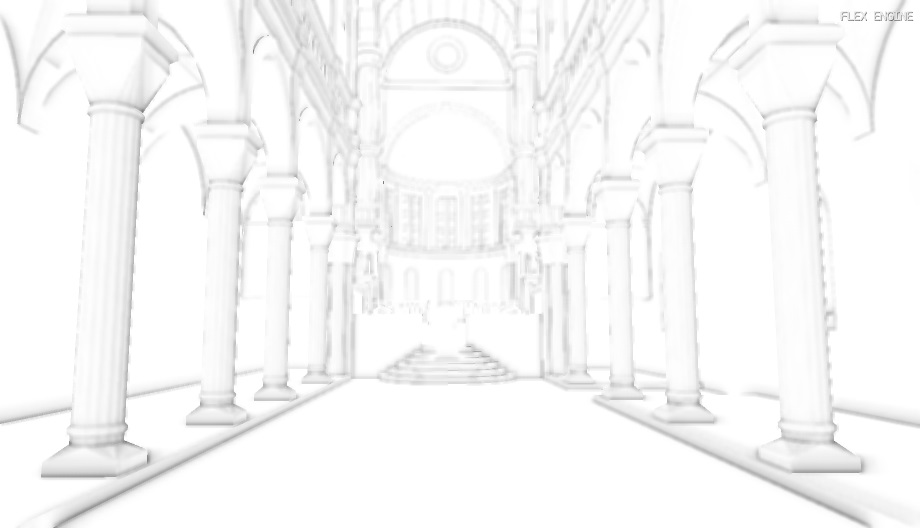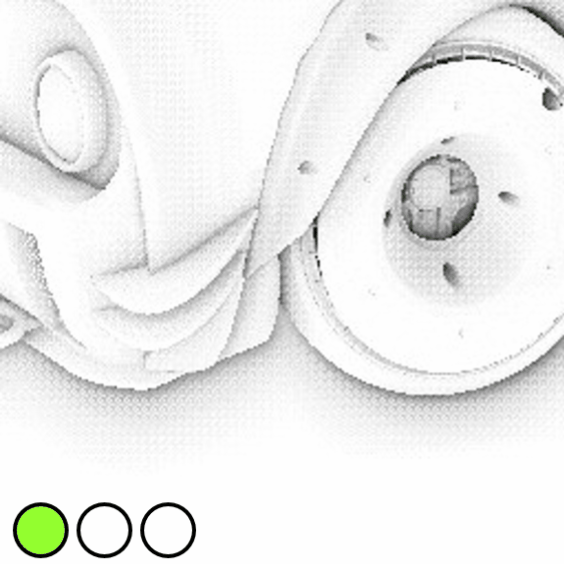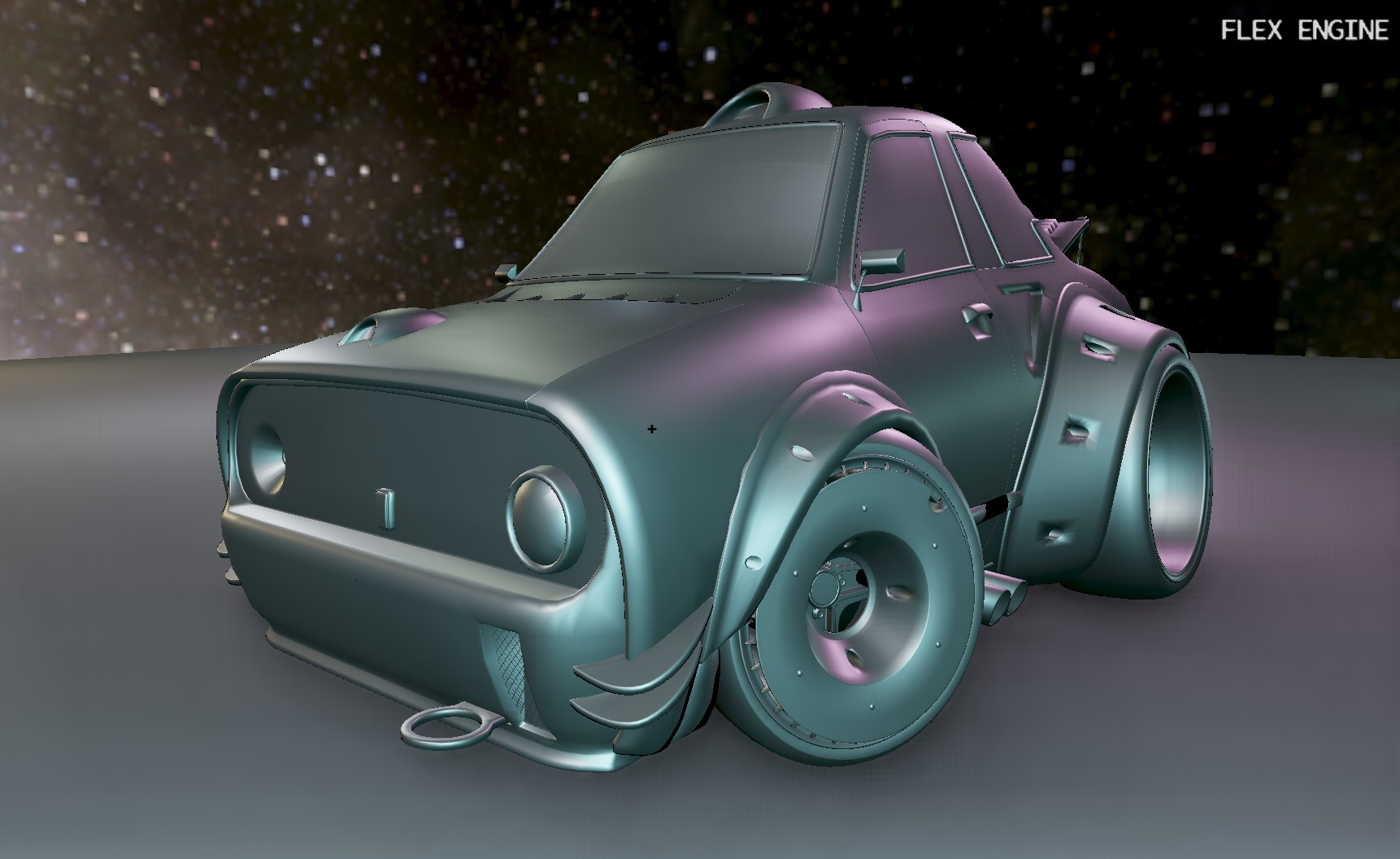SSAO (Screen-space ambient occlusion) is a widespread technique employed by many games to simulate the shadowing effect of objects occluding other nearby objects. It was originally proposed by Crytek in 2007 (original paper ), and has since seen many improvements. I’ve implemented a variant of it in Flex recently, and this is a short discussion of the implementation details.

The necessary inputs are the scene depth and normals, both in view space. The depth buffer will be used as a rough estimate of how occluded each pixel is, and the normals will be used to orient a hemisphere about each pixel to generate our sample points in. Because Flex already supports deferred rendering, these two input buffers were readily available. The only change necessary was to output the normals in view space, rather than world space.

Instead of depth, some implementations require a three-channel position buffer as input. I’ve opted to reconstruct position from the depth buffer instead to save on texture bandwidth. There’s a number of ways to do this, if you’re implementing this yourself then definitely give this three part series by MJP a read. I opted for using the already existing view-space hardware depth buffer. Here’s how I’m unpacking that into a view-space position:

``````vec3 reconstructVSPosFromDepth(vec2 uv)
{
float depth = texture(in_Depth, uv).r;
float x = uv.x * 2.0 - 1.0;
float y = (1.0 - uv.y) * 2.0 - 1.0;
vec4 pos = vec4(x, y, depth, 1.0);
vec4 posVS = uboConstant.invProj * pos;
return posVS.xyz / posVS.w;
}
``````

In order to avoid banding artifacts we will modulate the sample points by some random noise. A 4x4 noise texture can be generated at startup. To get random rotations around the z-axis, our texture will contain values in the range [-1.0, 1.0] in the red and green channels. This requires the use of a floating point texture format, but could easily be scaled and biased to fit into an integral format just as well.

Packed two channel noise textureTo orient the hemisphere about the normal, I first use the Gram-Schmidt process to obtain a local coordinate frame:

``````ivec2 depthSize = textureSize(in_Depth, 0);
ivec2 noiseSize = textureSize(in_Noise, 0);
float renderScale = 0.5; // SSAO is rendered at 0.5x scale
vec2 noiseUV = vec2(float(depthSize.x)/float(noiseSize.x),
float(depthSize.y)/float(noiseSize.y))
* ex_UV * renderScale;
vec3 randVec = texture(in_Noise, noiseUV).xyz;

vec3 tangent = normalize(randVec - normal * dot(randVec, normal));
vec3 bitangent = cross(tangent, normal);
mat3 TBN = mat3(tangent, bitangent, normal);
``````

Then the real meat of the algorithm can commence:

``````float bias = 0.01;

float occlusion = 0.0;
for (int i = 0; i < SSAO_KERNEL_SIZE; i++)
{
vec3 samplePos = TBN * uboConstant.samples[i].xyz;
samplePos = posVS + samplePos * SSAO_RADIUS;

// Convert view-space position into clip-space
vec4 offset = vec4(samplePos, 1.0);
offset = uboConstant.projection * offset;
offset.xy /= offset.w;
offset.xy = offset.xy * 0.5 + 0.5;
offset.y = 1.0 - offset.y;

vec3 reconstructedPos = reconstructVSPosFromDepth(offset.xy);
occlusion += (reconstructedPos.z <= samplePos.z - bias ? 1.0 : 0.0);
}
occlusion = 1.0 - (occlusion / float(SSAO_KERNEL_SIZE));

fragColor = occlusion;
``````

In essence, we count all points that are farther into the scene than the current fragment as occluders, and all others as non-occluders. Note that this is calculated at half-size since it a low-frequency effect and this quarters the execution time necessary.

This solution however has some gaping holes which need to be patched up. For one, large depth discontinuities are ignored, causing a dark halo effect around objects which are well in front of other objects. This can be addressed by scaling the occlusion factor based a point’s distance from the center.

Another big issue with the current implementation is the level of noise. We traded off banding artifacts for noise by jittering the sampled location, but a simple blur pass will greatly improve the final result. The blur should run at full-resolution in order to avoid cracks at the edges of objects. I first implemented a simple box blur, but later replaced it with a two-pass edge-preserving bilateral blur covered in more detail below.

``````float rangeCheck = smoothstep(0.0, 1.0, uboConstant.ssaoRadius / abs(reconstructedPos.z - samplePos.z - bias));
occlusion += (reconstructedPos.z <= samplePos.z - bias ? 1.0 : 0.0) * rangeCheck;
``````### Two-pass bilateral blur

An NxN box blur is a simple effect to implement, however also an expensive one to compute, especially as N increases. The technique is to simply average all the pixel values around the pixel you’re blurring (extending N pixels out).

A much more efficient approach is to use a separable blur. This just means computing the blur in two passes. The first pass will calculate the horizontal blur using just N samples, and the second will compute the vertical blur, again using just N samples. Because the blur filter is “separable”, this is mathematically-equivillent and therefore produces the same image. The reduction from N2 samples to just 2N samples is a huge win. The three stages of the bilateral blur are shown below.In order to avoid blurring across hard edges, the normal and depth buffers are passed into the blur shader. When sampling neighbouring pixels, samples whose depth lie outside of a given distance or whose normal differs by a large-enough value are ignored. This allows for edges to remain sharp, and prevents blurring across large depth discontinuities. This extra step makes the blur bilateral (or, “edge-preserving”).

### Specialization Constants

I utilized SPIR-V’s specialization constant feature to retrieve a unique ID for the kernelSize constant uniform so it can be updated without needing to modify the shader code dynamically. See  for a great explanation.Thanks for reading! As always, the source code is all available on GitHub.

Thanks to luyssport for the car model!